Transforming Numerical Methods Education for the STEM Undergraduate MOOC | MOBILE | VIDEOS | BLOG | YOUTUBE | TWITTER | COMMENTS | ANALYTICS | ABOUT | CONTACT | COURSE WEBSITES | BOOKS | MATH FOR COLLEGE
 MULTIPLE CHOICE TEST GAUSS-SEIDEL METHOD SIMULTANEOUS LINEAR EQUATIONS

Q1. A square matrix [A]nxn is diagonally dominant if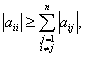i = 1, 2, …, n= 1, 2, …, n  and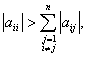for any i  = 1, 2, …, n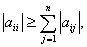i = 1, 2, …, and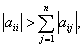for any i = 1, 2, …, ni = 1, 2, …, n

Q2. Using [x1   x2   x3] = [1   3   5] as the initial guess, the value of [x1   x2   x3] after three iterations of Gauss-Seidal method is[-2.8333    -1.4333     -1.9727]

[1.4959     -0.90464    -0.84914]

[0.90666   -1.0115      -1.0242]

[1.2148     -0.72060    -0.82451]

Q3. To ensure that the following system of equations,converges using the Gauss-Siedal method, one can rewrite the above equations as follows:The equations cannot be rewritten in a form to ensure convergence.

Q4. Forand using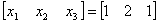as the initial guess, the values of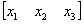are found at the end of each iteration as

 Iteration # x1 x2 x3 1 0.41666 1.1166 0.96818 2 0.93989 1.0183 1.0007 3 0.98908 1.0020 0.99930 4 0.99898 1.0003 1.0000

At what first iteration number would you trust at least 1 significant digit in your solution?

1

2

3

4

Q5. The algorithm for the Gauss-Seidal method to solve [A] [X] = [C] is given as follows when using nmax iterations. The initial value of [X] is stored in [X].

Sub Seidal(n, a, x, rhs, nmax)

For k = 1 To nmax

For i = 1 To n

For j = 1 To n

If (i <> j) Then

Sum = Sum + a(i, j) * x(j)

endif

# Next j

x(i) = (rhs(i) - Sum) / a(i, i)

Next i

Next k

End Sub

Sub Seidal(n, a, x, rhs, nmax)

For k = 1 To nmax

For i = 1 To n

Sum = 0

For j = 1 To n

If (i <> j) Then

Sum = Sum + a(i, j) * x(j)

endif

# Next j

x(i) = (rhs(i) - Sum) / a(i, i)

Next i

Next k

End Sub

Sub Seidal(n, a, x, rhs, nmax)

For k = 1 To nmax

For i = 1 To n

Sum = 0

For j = 1 To n

Sum = Sum + a(i, j) * x(j)

# Next j

x(i) = (rhs(i) - Sum) / a(i, i)

Next i

Next k

End Sub

Sub Seidal(n, a, x, rhs, nmax)

For k = 1 To nmax

For i = 1 To n

Sum = 0

For j = 1 To n

If (i <> j) Then

Sum = Sum + a(i, j) * x(j)

endif

# Next j

x(i) = rhs(i)  / a(i, i)

Next i

Next k

End Sub

Q6.  Thermistors measure temperature, have a nonlinear output and are valued for a limited range.  So when a thermistor is manufactured, the manufacturer supplies a resistance vs. temperature curve.  An accurate representation of the curve is generally given by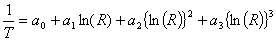where T is temperature in Kelvin, R is resistance in ohms, and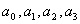are constants of the calibration curve.

Given the following for a thermistor

 R T ohm 1101.0 911.3 636.0 451.1 25.113 30.131 40.120 50.128

the value of temperature in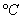for a measured resistance of 900 ohms most nearly is

30.002

30.472

31.272

31.445

 AUDIENCE |  AWARDS  |  PEOPLE  |  TRACKS  |  DISSEMINATION  |  PUBLICATIONS Copyrights: University of South Florida, 4202 E Fowler Ave, Tampa, FL 33620-5350. All Rights Reserved. Questions, suggestions or comments, contact kaw@eng.usf.edu  This material is based upon work supported by the National Science Foundation under Grant#0126793, 0341468, 0717624,  0836981, , 0836805, 1322586.  Any opinions, findings, and conclusions or recommendations expressed in this material are those of the author(s) and do not necessarily reflect the views of the National Science Foundation.  Other sponsors include Maple, MathCAD, USF, FAMU and MSOE.  Based on a work at http://mathforcollege.com/nm.  Holistic Numerical Methods licensed under a Creative Commons Attribution-NonCommercial-NoDerivs 3.0 Unported License. ANALYTICS